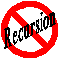Test IMG SRC: /images/recursion.gif
Test IMG:Test IMG:Test Define: The act of changing the order of elements arranged in a particular order, as abc into acb, bac, etc., or of arranging a number of elements in groups made up of equal numbers of the elements in different orders, as a and b in ab and ba; a one-to-one transformation of a set with a finite number of elements.permutation
Test Char: Θ
Θ Theta < ISO-8859-1
```				```
// NOTICE:  Copyright 1991-2008, Phillip Paul Fuchs
#define N    12  // number of elements to permute.  Let N > 2

void QuickPerm(void) {
unsigned int a[N], p[N+1];
register unsigned int i, j, tmp; // Upper Index i; Lower Index j

for(i = 0; i < N; i++) {  // initialize arrays; a[N] can be any type
a[i] = i + 1;  // a[i] value is not revealed and can be arbitrary
p[i] = i;
} // for(i < N)

p[N] = N;  // p[N] > 0 controls iteration and the index boundary for i
i = 1;  // setup first swap points to be 1 and 0 respectively (i & j)

while(i < N) {
p[i]--;            // decrease index "weight" for i by one
j = i % 2 * p[i];  // IF i is odd then j = p[i] otherwise j = 0
tmp = a[j];        // swap(a[j], a[i])
a[j] = a[i];
a[i] = tmp;
i = 1;             // reset index i to 1 (assumed)
while (!p[i]) {    // while (p[i] == 0)
p[i] = i;      // reset p[i] zero value
i++;           // set new index value for i (increase by one)
} // while(!p[i])
} // while(i < N)
}
```
```